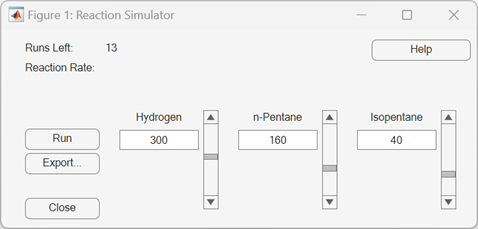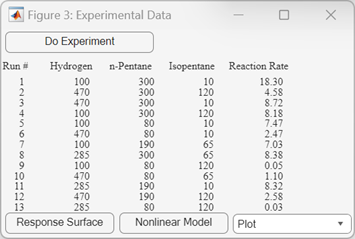# rsmdemo

Interactive response surface demonstration

## Syntax

```rsmdemo ```

## Description

`rsmdemo` opens a group of three graphical user interfaces for interactively investigating response surface methodology (RSM), nonlinear fitting, and the design of experiments.

The interfaces allow you to collect and model data from a simulated chemical reaction. Experimental predictors are concentrations of three reactants (hydrogen, n-pentane, and isopentane) and the response is the reaction rate. The reaction rate is simulated by a Hougen-Watson model (Bates and Watts, , pp. 271–272):

`$rate=\frac{{\beta }_{1}{x}_{2}-{x}_{3}/{\beta }_{5}}{1+{\beta }_{2}{x}_{1}+{\beta }_{3}{x}_{2}+{\beta }_{4}{x}_{3}}$`

where rate is the reaction rate, x1, x2, and x3 are the concentrations of hydrogen, n-pentane, and isopentane, respectively, and β1, β2, ... , β5 are fixed parameters. Random errors are used to perturb the reaction rate for each combination of reactants.

Collect data using one of two methods:

1. Manually set reactant concentrations in the Reaction Simulator interface by editing the text boxes or by adjusting the associated sliders.When you click , the concentrations and simulated reaction rate are recorded on the Trial and Error Data interface.You are allowed up to 13 independent experimental runs for data collection.

2. Use a designed experiment to set reactant concentrations in the Experimental Data interface by clicking the button.A 13-run D-optimal design for a full quadratic model is generated by the `cordexch` function, and the concentrations and simulated reaction rates are recorded on the same interface.Once data is collected, scatter plots of reaction rates vs. individual predictors are generated by selecting one of the following from the Plot pop-up menu below the recorded data:

• Hydrogen vs. Rate

• n-Pentane vs. Rate

• Isopentane vs. Rate

Fit a response surface model to the data by clicking the button below the trial-and-error data or the button below the experimental data. Both buttons load the data into the Response Surface Tool `rstool`. By default, trial-and-error data is fit with a linear additive model and experimental data is fit with a full quadratic model, but the models can be adjusted in the Response Surface Tool.

For experimental data, you have the additional option of fitting a Hougen-Watson model. Click the button to load the data and the model in `hougen` into the Nonlinear Fitting Tool `nlintool`.